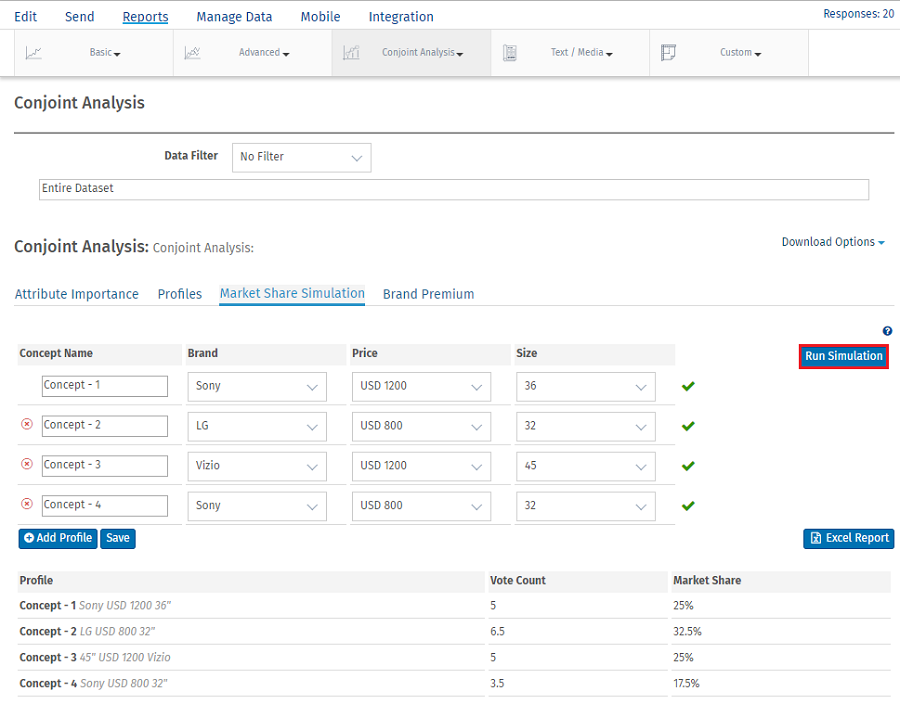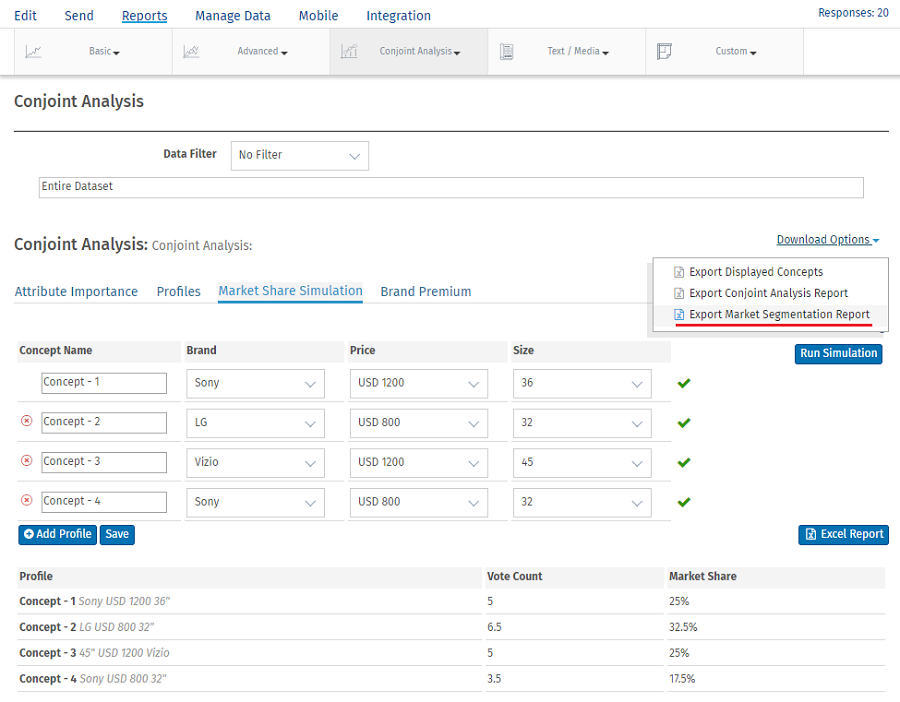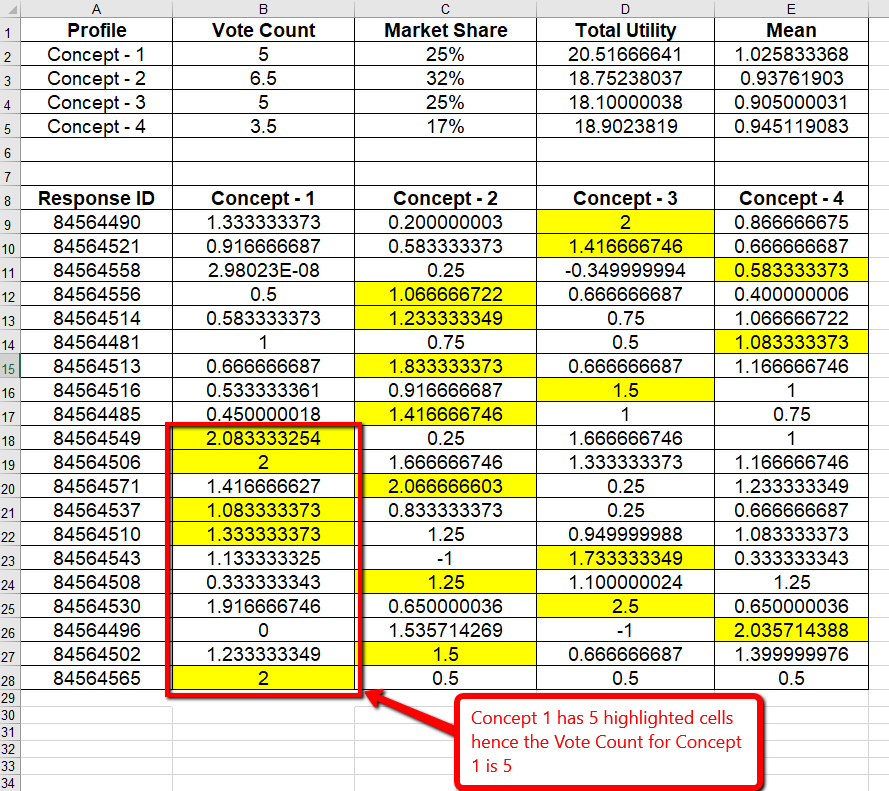# Market Segmentation Simulator

The Conjoint Simulator is by far the most interesting aspect of Conjoint Analysis. This gives you the ability to "predict" the market share of new products and concepts that may not exist today. This also gives you the ability to measure the "Gain" or "Loss" in market share based on changes to existing products in the given market.

The first step in Conjoint Simulation is identifying and describing the different products or concepts that you want to investigate. We call these "Profiles". As mentioned earlier, any product or concept can be identified or created using the Attributes and Levels that have been defined for the study. For the purposes of the Conjoint Simulator, a product or concept comprises of all the Attributes and one level from each of the attributes.

For example one of the profiles could be: Brand - Sony, Price - \$899, Size - 42"

In most cases we would want to find out all the existing products that are available in that market segment and simulate the market share of the products to establish a baseline. Once this is established, it would be a good starting point for trying out new services and ideas and see how the market share shifts based on new products and configurations.

To view detailed Market Share Simulation:

Go to: Login » Surveys » Reports » Choice Modelling » Conjoint Analysis » Market Share Simulation

Add profile as shown below and click on Run Simulation button. This provides the report with Vote Count and Market Share of respective concept.Calculations for Vote CountSince in the example we added 4 concepts we have part worth value for 4 concepts.

Here the Utility value is derived from the following formula:

Utility Value = Σlog(e (W * Selected Configuration) / e (W * All Configurations))

• Where W is one-dimensional part-worth array.
If we have A attributes, a = 1 to A, with each attribute having La levels, l = 1 to La, then the part-worth for a particular attribute/level is w’(a,l). It is this (jagged array) of part-worths we are solving for in this exercise.
We can simplify this to a one-dimensional array w(s), where the elements are:{w’(1,1), w’(1,2) ... w’(1,L1), w’(2,1) ... w’(A,LA)} with w having S elements.
• Selected Configuration(levels selected in concept) is in form of array where selected= 1.
• All configuration(levels appeared in concept) is also in form of an array.
• We then take the dot product of (W * Selected Configuration) and (W * All Configurations) and impute in the mentioned formula to get the utility values.

We calculate the max of Utility value between 4 concepts row wise.In the above example max Utility value between 4 concepts are highlighted in yellow.

We then take the count of these highlighted cells which gives us vote count.If there is any tie then we take average.

In the above example, Concept 1 has 5 highlighted cells hence the Vote Count is 5.

Concept 2 has 7 highlighted values of which Response Id : 84564508 has two max values 1.25. In such tie situation we average out by ½ =0.5. (If 1.25 was present in one more concept than average would be ⅓ = 0.33) . Hence the Vote Count will be 6.5.

Likewise Vote Count is Calculated for Concept 3 and 4.

We take the Total of Vote Count = 5+6.5+5+3.5 = 20

Market Share = Individual Vote Count/Total Vote Count *100

Market Share of Concept 1 = (5/20)*100 = 25%
Market Share of Concept 2 = (6.5/20)*100 = 32.5%
Market Share of Concept 3 = (5/20)*100 = 25%
Market Share of Concept 4 = (3.5/20)*100 = 17.5%

This feature/tools described here are available with the following license(s) :

Enterprise Edition

Unlimited Surveys, Questions